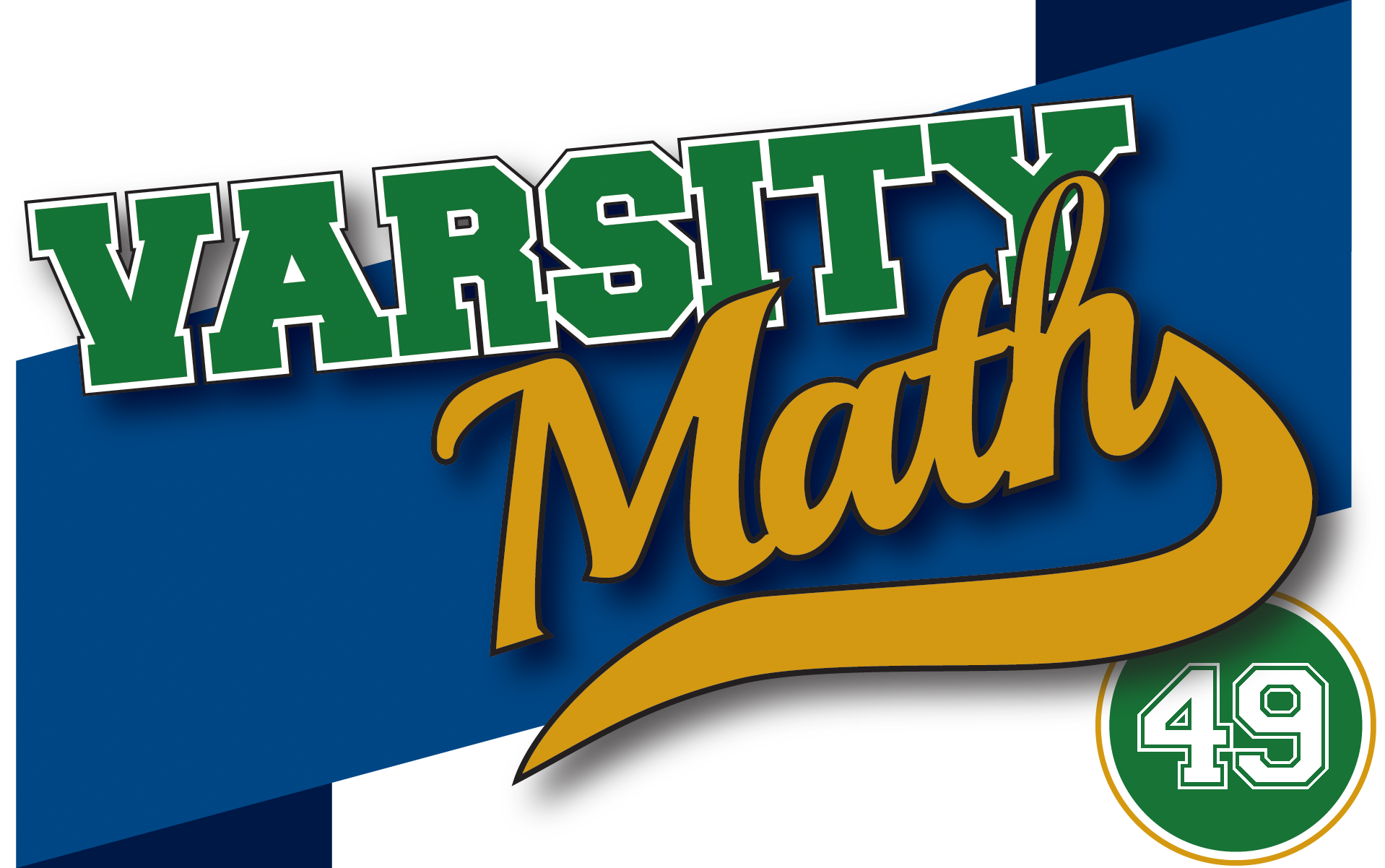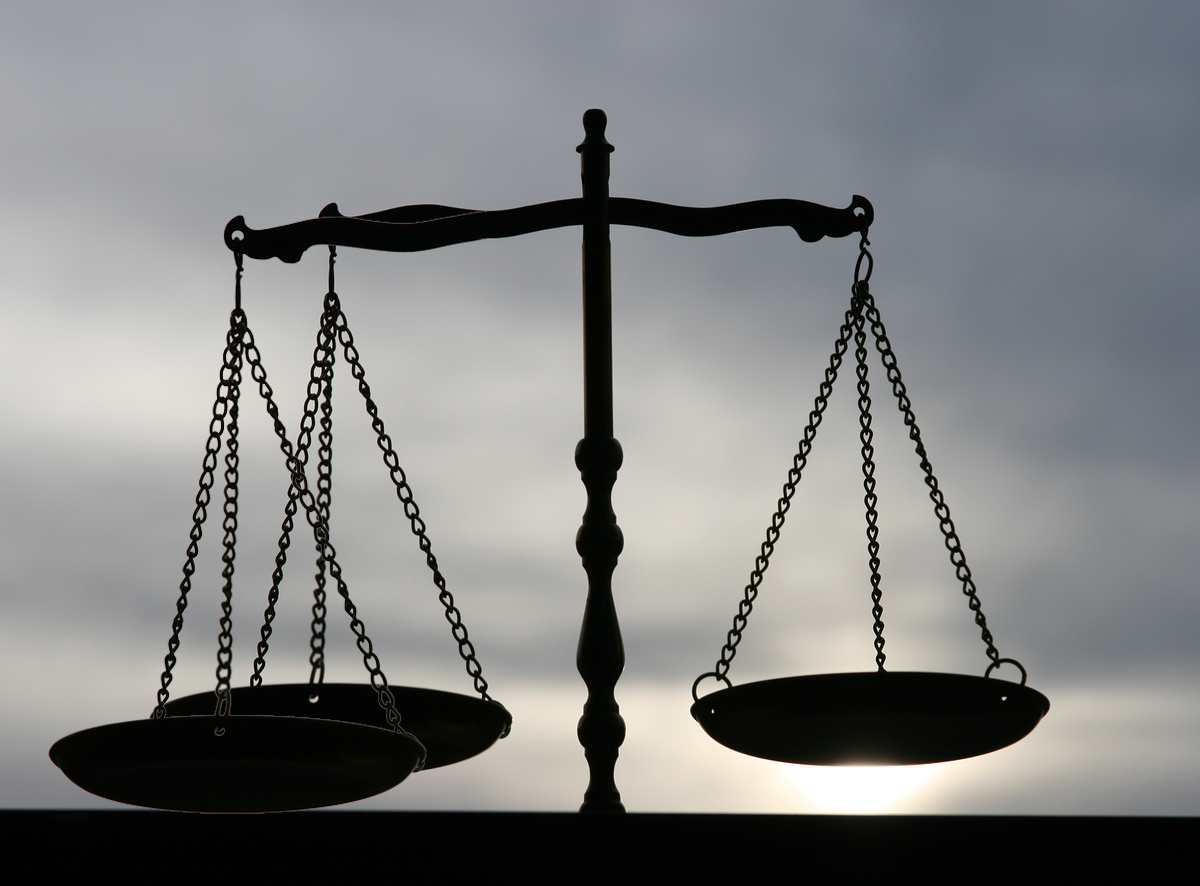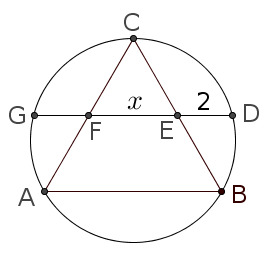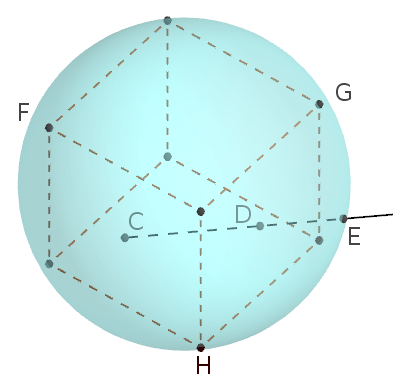## ________________

Summer vacation has allowed the team members some time for tinkering.

## ________________### Three-Pan Alley

“Eureka!” shouts Harper. “Ever since that Old Coin Game a couple months ago, I have been working on my three-pan balance, and I have almost perfected it. You put objects in each of the three pans, and you can tell if they all weigh the same, or if two weigh the same and one is different — in which case you can tell which it is and whether it is lighter or heavier. Right now it produces unpredictable results when the three pans all hold different weights, but even so, I can detect a counterfeit among more coins in two weighings than you can in three weighings with a regular balance.” Recall, the setup is that you get a certain number of coins, including a specific one that’s guaranteed to be real. The set of coins may have one counterfeit coin, which could be either lighter or heavier. The goal is to determine if there is a counterfeit and if so, which one it is and whether it is lighter or heavier.

What is the greatest number of coins among which Harper can always detect a counterfeit in just two weighings with the three-pan balance?

### Urn More Respect

“That’s nothing,” responds Kai. “I’ve been working on my exchanging urns. They’re two linked urns, and after every time you draw one ball from either urn, assuming there’s at least one ball left in each urn, a random ball from one urn exchanges places with a random ball from the other urn. That gives us all kinds of new problems! For example, suppose I start with two red balls and one blue one in one urn, and one red and two blues in the other, but you don’t know which. You pick an urn, draw out a ball, and it’s red. Then you draw a second ball from the same urn (without putting the first one back). It’s also red. With ordinary urns, you’d know that was the urn that started with two red balls. But with my exchanging urns, you don’t!”

In the sequence of events described, what is the probability that the urn from which you drew the balls is the one that started with two reds?

## Solutions to week 48Circular Triangle. Let x be the requested length of segment EF, as shown in the diagram. Since ABC is equilateral, in order for AE and BF to be the angle bisectors of angles A and B, points E and F must be the midpoints of their respective sides. Therefore, triangle CEF is an isosceles triangle with apex angle 60°, and so it is equilateral. Therefore, segments FC, CE, and EB all have length x. Also, by symmetry, segment FG has length two.

But now the power of the point E in the circle is CE · EB = DE · EG, so x · x = 2(x + 2). Solving x² – 2x – 4 = 0 yields x = 1 ± √5. Since segment lengths must be positive, we conclude that the length of segment EF is 1 + √5.Spherical Cube. Let the point where the ray r from C to D crosses the sphere be called E, as shown in the diagram. The problem asks for length DE. First, let’s compute length CD. Projecting down onto the bottom face of the cube, we see that CD is just the hypotenuse of an isosceles right triangle with legs equal to 1/2, and hence it has length 1/√2. Now, to find the length DE, consider vertices F, G, and H of the cube as shown. Of course face diagonals FG, GH, and HF are equal. Also, C is the midpoint of HF and D is the midpoint of GH. Consider the plane defined by points F, G, and H. That plane intersects the sphere in a circle (since all planes do), and certainly F, G, and H are on this circle. Since C and D are also in this plane, ray r is as well, and therefore E lies in this plane, on the circle containing F, G, and H. In other words, restricting ourselves to this plane, the diagram for this problem is identical to the previous one! (with F, G, and H playing the roles of A, B, and C, respectively.) Since in the previous problem, DE was 2/(1 + √5) as long as EF (the reciprocal of the golden ratio!), we know that in this problem DE is 2/(1 + √5) · 1/√2 = √2 / (1 + √5).